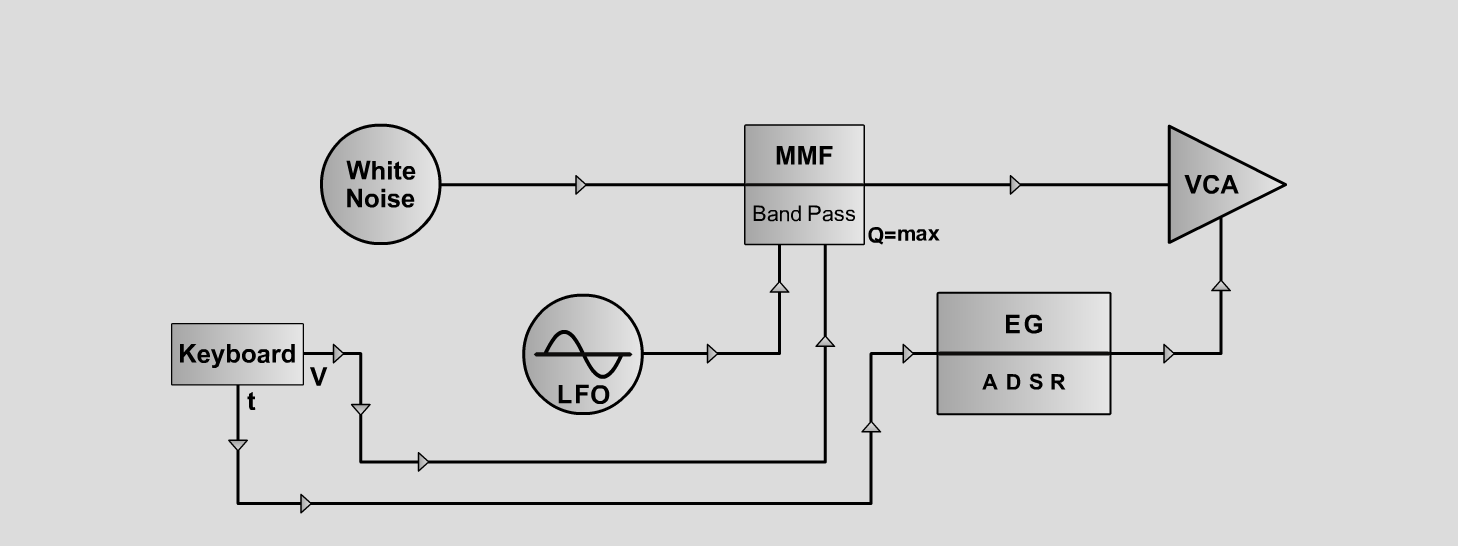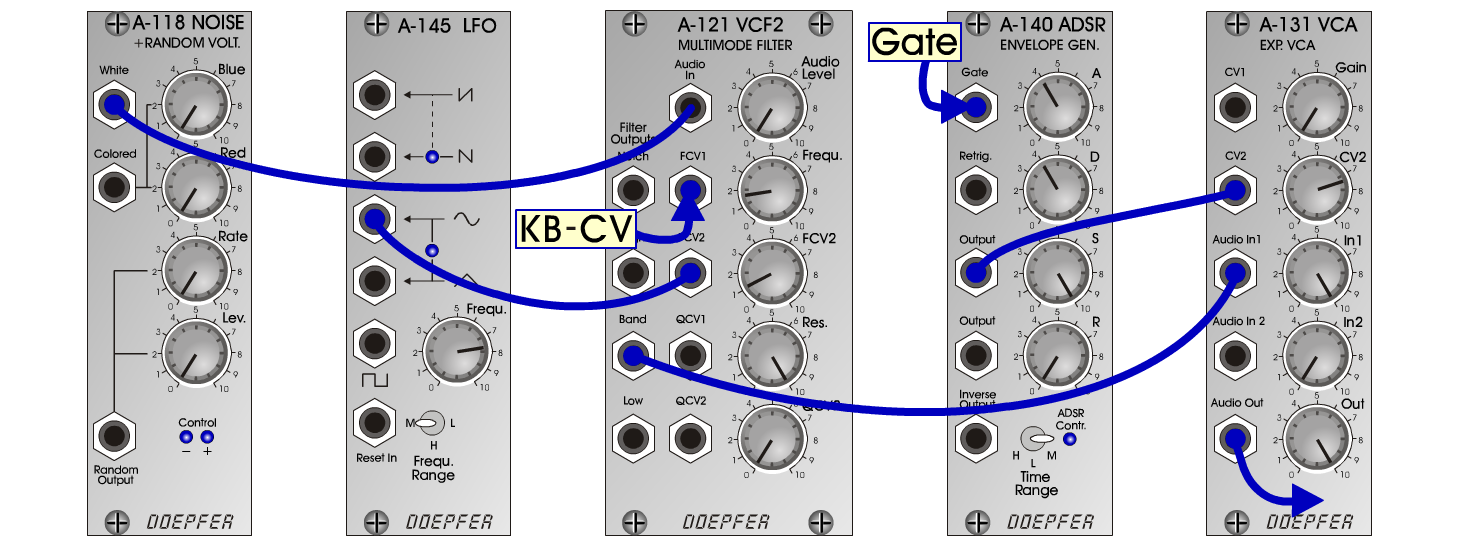D o e p f e r A - 100 Connections: Settings: A-118 (White) <=> A-121 (Audio In)  A-121 (Band) <=> A-131 (Audio In)  A-145 (Sine) <=> A-121 (FCV2)  A-140 (Output) <=> A-131 (CV2)  KB-CV <=> A-121 (FCV1) A-121 (Audio Level = 0, Freq = 1.7, FCV 2 = 1.1, Res = 10)  A-145 (Frq = 7.7, Range = M)  A-140 (A = 4, D = 4, S = 10, R = 0, Range = M)  A-131 (Gain = 0, CV2 = 7.4, Audio In 1 = 10, Audio Out = 10) Ghost B: A-121 (Audio Level = 5) Josef MuellerSound samples Bach - So nun der Geist ((BWV 227))     2. sound: E-Organ Ghost A 1 Ghost A 2 Ghost A 3 Ghost B 1 Ghost B 2 Ghost B 3